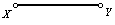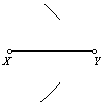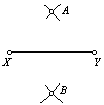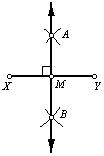Construct the perpendicular bisector of a line segment.

Or, construct the midpoint of a line segment.

 1. Begin with line segment XY.2. Place the compass at point X. Adjust the compass radius so that it is more than (1/2)XY. Draw two arcs as shown here.3. Without changing the compass radius, place the compass on point Y. Draw two arcs intersecting the previously drawn arcs. Label the intersection points A and B.4. Using the straightedge, draw line AB. Label the intersection point M. Point M is the midpoint of line segment XY, and line AB is perpendicular to line segment XY.Back to Geometry Construction Reference